# NCERT Solutions for Class 10 Science Chapter 13 Magnetic Effects of Electric Current

NCERT Solutions for Class 10 Science Chapter 13 Magnetic Effects of Electric Current

NCERT Questions

In Text Questions

Question 1.
Why does a compass needle get deflected when brought near a bar magnet ?
Compass needle is a small magnet which experiences a force in the magnetic field of a bar magnet. Due to this force, it gets deflected.

Question 2.
Draw magnetic field lines around a bar magnet. (CBSE 2011, 2012, 2013, 2014)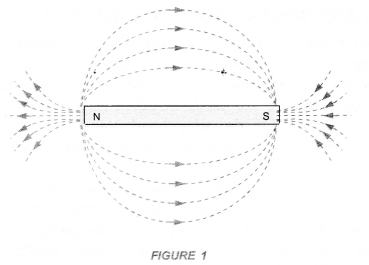Question 3.
List the properties of magnetic field lines. (CBSE 2015)
Or
Write four properties of magnetic field lines. (CBSE (Delhi) 2010, 2011, 2012, 2013)

1. Magnetic field lines are closed continuous curves.
2. The tangent at any point on the magnetic field line gives the direction of the magnetic field at that point.
3. No two magnetic field lines can cross each other.
4. Magnetic field lines are crowded in a region of strong magnetic field and magnetic field lines diverge in a region of weak magnetic field.

Question 4.
Why do not two magnetic field lines intersect each other ? (CBSE 2011, 2012, 2015)
Or
No two magnetic field lines can intersect each other. Explain. (CBSE 2010, 2014)
The tangent at any point on a magnetic field line gives the direction of magnetic field at that point. If two magnetic field lines cross each other, then at the point of intersection, there will be two tangents. Hence, there will be two directions of the magnetic field at the point of intersection. This is not possible. Hence, no two magnetic field lines can cross each other.Question 5.
Consider a circular loop of wire lying in the plane of the table. Let the current pass through the loop clockwise. Apply the right hand rule to find out the direction of magnetic field inside and outside the loop.
(CBSE (Delhi) 2009)
Magnetic field inside the loop is perpendicular to the plane of table and in the downward direction. However, outside the loop, magnetic field is perpendicular to the plane of the table and in the upward direction.

Question 6.
The magnetic field in a given region is uniform. Draw a diagram to represent it.
(CBSE 2011, 2012, 2015)Question 7.
Choose the correct option :
The magnetic field inside a long straight solenoid carrying current is :
(a) zero
(b) decreases as we move towards its ends .
(c) increases as we move towards its ends
(d) is the same at all points.
(b).

Question 8.
Which of the property a proton can change when it moves freely in a magnetic field ? (There may be more than one correct answer).
(a) mass
(b) speed
(c) velocity
(d) momentum.
A force acts on a proton when it moves freely in a magnetic field. Hence its velocity and momentum can change.

Question 9.
In activity 13-7 (NCERT book), how do we think the displacement of rod AB will be affected if

1. current in rod AB is increased
2. a stronger horse shoe magnet is inserted
3. length of the rod AB is increased.

Force acting on a current carrying conductor of length l placed perpendicular to magnetic field B is given by F = B I l

1. When I increase, F also increases. Hence the displacement of the rod increases.
2. When a stronger horse shoe magnet is inserted, magnetic field at B increases. So force also increases. Hence displacement increases.
3. When l increases, force increases and hence displacement increases.

Question 10.
A positively charged partical (alpha partical) projected towards west is deflected towards north by a magnetic field. The direction of magnetic field is
(a) towards south
(b) towards east
(c) downward
(d) upward.
(d).

Question 11.
State Fleming’s left hand rule with a labelled diagram. (CBSE 2005, 2010, 2011, 2012)
Fleming’s left hand rule
Statement : Stretch the left hand such that the thumb, first finger and the central finger are mutually perpendicular to each other. If the First finger points in the direction of the magnetic Field and the Central finger points in the direction of Current, then the thumb will point in the direction of Motion (or Force) as shown in figure 24.Thus, in Fleming’s left hand rule, first finger shows the direction of the magnetic field. The central finger shows the direction of electric current flowing in the conductor. Thumb shows the direction of force on the conductor or the direction of motion of the conductor

Question 12.
What is the principle of electric motor ? (CBSE 2012)
Electric motor works on the principle that a current carrying conductor placed perpendicular to a magnetic field experiences a force.

Question 13.
What is the role of the split ring in an electric motor ?
The split-ring in an electric motor reverses the direction of current in the armature coil of the motor. Therefore, the direction of the force acting on the two arms of the coil is also reversed. As a result of this, the coil of d.c. motor continues to rotate in the same direction.

Question 14.
Explain different ways to induce current in a coil. (CBSE 2010, 2011, 2012)

1. By moving a bar magnet towards or away from the coil.
2. By placing a closed coil near another coil connected across a battery.

Question 15.
State the principle of electric generator.
It is based on the principle of electromagnetic induction. That is, the changing magnetic field induces current in the coil.

Question 16.
Name some sources of direct current. (Bihar Board 2012, 2015)
A dry cell, a battery, a solar cell, d.c. generator etc. are some sources of direct current.

Question 17.
Which source produces alternating current ?
AC generator (which converts mechanical energy into alternating current or electricity) and an oscillator (a device which converts D.C. into A.C.) are the sources which produce alternating current.

Question 18.
Choose the correct option :
A rectangular coil of copper wires is rotated in a magnetic field. The direction of the induced current changes once in each
(a) two revolutions
(b) one revolution
(c) half revolution
(d) one-fourth revolution,
(c).

Question 19.
Name two safety measures commonly used in electric circuit and appliances.
(CBSE 2010, 2012, 2014, 2015)

1. Electric fuse and
2. earthing.

Question 20.
An electric oven of 2 kW power rating is operated in a domestic electric circuit (220 V) that has a current rating of 5 A. What result do you expect ? Explain. (CBSE 2010, 2011, 2012, 2013, 2014)
P = 2 kW = 2000 W and V = 220 VThis shows that current flowing through the oven is more than the current rating (5 A). Hence, the fuse in the circuit melts and oven is saved from damage.

Question 21.
What precautions should be taken to avoid the overloading of domestic electric circuit ? (CBSE 2010, 2011, 2012, 2015)
Or
Write any two precautions to be taken to avoid overloading of a domestic electric circuit.

1.  We should not connect many appliances in the same socket.
2. Electrical appliances of high power rating should not be switched on simultaneously.

NCERT Chapter End Exercises

Question 1.
Which of the following correctly describes the magnetic field near a long straight wire ?
(a) the field consists of straight lines perpendicular to the wire
(b) the field consists of straight lines parallel to the wire
(c) the field consists of radial lines originating from the wire
(d) the field consists of concentric circles centered on the wire.
(d).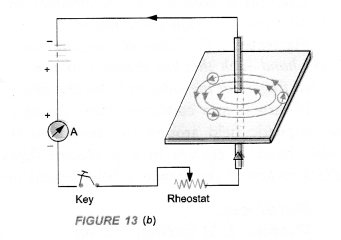Question 2.
The phenomena of electromagnetic induction is
(a) the process of charging a body
(b) the process of generating magnetic field due to current passing through a coil
(c) producing induced current in a coil due to relative motion between a magnet and the coil
(d) the process of rotating a coil of an electric motor.
(c).

Question 3.
The device used for producing electric current is called a
(a) generator
(b) galvanometer
(c) ammeter
(d) motor. (Bihar Board 2012)
(a).

Question 4.
The essential difference between an AC generator and a DC generator is that
(a) AC generator has an electromagnet while a DC generator has permanent magnet
(b) DC generator will generate a higher voltage
(c) AC generator will generate & higher voltage
(d) AC generator has slip rings while the DC generator has a commutator.
(d).

Question 5.
At the time of short circuit, the current in the ciruit
(a) reduces substantially
(b) does not change
(c) increases heavily
(d) vary continuously. (Bihar Board 2012)
(c).

Question 6.
State whether the following statements are true or false :
(a) an electric motor converts mechanical energy into electrical energy ‘
(b) an electric generator works on the principle of electromagnetic induction
(c) the field at the centre of a long circular coil carrying current will be parallel straight lines
(d) a wire with a green insulation is usually the live wire.
(a) False. It converts electrical energy into mechanical energy.
(b) True.
(c) True.
(d) False. Five wire has red insulation cover.

Question 7.
List three sources of magnetic fields. (CBSE 2013)

1. a permanent magnet
2. a current carrying conductor
3. a current carrying solenoid.

Question 8.
How does a solenoid behave like a magnet ? Can you determine the north and south poles of a current carrying solenoid with the help of a bar magnet. Explain. (CBSE 2012, 2013)
When electric current flows through a solenoid, magnetic field is set up around the solenoid. The pattern of the magnetic field is same as that of the magnetic field of a bar magnet. One end of the solenoid behaves as north pole and the other end of the solenoid behaves as south pole.
To determine the north and south poles of a current carrying solenoid with the help of a bar magnet, suspend it with a strong thread. Now bring the north pole of a bar magnet towards one end of the solenoid. If the solenoid attracts towards the magnet, then that face of the solenoid is south pole. If the bar magnet moves away from the solenoid, then that face of the solenoid is the north pole.

Question 9.
When is the force experienced by a current carrying conductor placed in magnetic field is the largest ?
[CBSE, (All India) 2009, 2010]
Or
Under what condition does a current carrying conductor kept in a magnetic field experience maximum force ? (CBSE 2011, 2012, 2014, 2015)
When current carrying conductor is placed perpendicular to the magnetic field.

Question 10.
Think you are sitting in a chamber with your back to one wall. An electron beam moving horizontally from back wall towards the front wall, is deflected by a strong magnetic field to your right side. What is the direction of magnetic field ?
Movement from electron beam from back wall to the front wall is equivalent to the flow of electric current from front wall to the back wall. The deflection of the beam means, the force is acting towards our right side. According to Fleming’s Left Hand Rule, the direction of magnetic field is vertically downward. That is, the magnetic field is perpendicular to the plane of the paper and directed inward.
Such magnetic field is shown by ⊗.Question 11.
Draw a labelled diagram of an electric motor. Explain its principle and working. What is the function of a split ring in an electric motor ?
Electric motor converts electrical energy into mechanical energy.
Principle: Electric Motor is based on the fact that a current carrying conductor placed perpendicular to the magnetic field experiences a force.1. Armature coil: It consists of a single loop of an insulated copper wife in the form of a rectangle. Rectangle ABCD shown in figure 25 is an armature coil.2. Strong field magnet: Armature coil is placed between two pole pieces (N and S poles) of a strong magnet. This magnet provides a strong magnetic field.
3. Split-ring type Commutator. It consists of two halves (R1 and R2) of a metallic ring. The two ends of the armature coil are connected to these two halves of the ring. Commutator reverses the direction of current in the armature coil.
4. Two carbon brushes B1 and B2 press against the commutator. These brushes act as the contacts between the commutator and the terminals of the battery.
5. A battery is connected across the carbon brushes. This battery supplies the current to the armature coil.

Question 12.
Name some devices in which electric motors are used.
Electric cars, rolling mills, electric fans, hair dryers, mixers, blenders etc.

Question 13.
A coil of insulated copper wire is connected to a galvanometer. What will happen if a bar magnet is

1. pushed into the coil,
2. withdrawn from inside the coil,
3. held stationary inside the coil ?

Name the phenomenon involved in the above cases.
(CBSE 2010, Term I, 2011, 2012, 2013, 2014)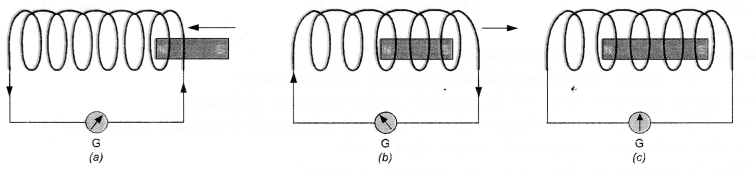1. When a bar magnet is pushed into the coil, induced current flows through the coil due to the phenomenon of electromagnetic induction. This induced current is indicated by the deflection of the needle of the galvanometer as shown in figure (a).
2. When a bar magnet is withdrawn from inside the coil, again induced current flows through the coil due to the phenomenon of electromagnetic induction. In this case, the direction of induced current is opposite to the direction of the current in case (i) as shown in figure (b).
3. When the bar magnet is held stationary inside the coil, there is no change in magnetic field around the coil. Hence, no induced current flows through the coil. Therefore, galvanometer shows no deflection as shown in figure (c).
Phenomenon involved in electromagnetic induction.

Question 14.
Two circular coils A and B are placed close to each other. If the current in the coil A is changed, will some current be induced in the coil B 1 Give reason. (CBSE 2010, 2012)
When current in coil A is changed, a changing magnetic field is set up around it. This changing magnetic field also links with coil B and hence some current will be induced in coil B due to electromagnetic induction.

Question 15.
State the rule to determine the direction of a

1. magnetic field produced around a straight conductor carrying current,
2. force experienced by a current-carrying straight conductor placed in a magnetic field which is perpendicular to it, and
3. current induced in a coil due to its rotation in a magnetic field.
(CBSE 2010, 2011, 2014)

1. Right hand thumb rule,
2. Fleming’s left hand rule,
3. Fleming’s right hand rule.

Question 16.
Explain the underlying principle and working of an electric generator by drawing a labelled diagram. What is the function of brushes ?
An electric device used to convert mechanical energy (kinetic energy) into electrical energy (electricity) is called an electric generator.
Principle: Electric generator works on the principle of electromagnetic induction. When the coil of electric generator rotates in a magnetic field, induced current flows in the circuit connected with the coil.
types of electric generator

1. AC generator
2. DC generator

AC generator: AC generator converts mechanical energy into electrical energy in the form of alternating current or AC.
DC generator: DC generator converts mechanical energy into electrical energy in the form of direct current or DC.                                                AC Generator Construction : The main components of AC generator are (Figure 33) :1. Armature : Armature coil (ABCD) consists of large number of turns of insulated copper wire wound over a soft iron core.
2. Strong field magnet : A strong permanent magnet or an electromagnet whose poles (N and S) are cylindrical in shape is a field magnet. The armature coil rotates between the pole pieces of the field magnet. The uniform magnetic field provided by the field magnet is perpendicular to the axis of rotation of the coil.
3. Slip Rings : The two ends of the armature coil are connected to two brass slip rings R1 and R2. These rings rotate along with the armature coil. Rings R1 and R2 are at different heights.
4. Brushes : Two carbon brushes (B1 and B2), are pressed against the slip rings. The brushes are fixed while slip rings rotate along with the” armature. These brushes are connected to the external circuit across which the output is obtained.

Working : When the armature coil ABCD rotates in the magnetic field provided by the strong field magnet, it cuts the magnetic field lines. Thus, the changing magnetic field produces induced current in the coil. The direction  of the induced current in the coil is determined by the Fleming’s right hand rule.
The current flows out through the brush B1 in one direction in the first half of the revolution and through the brush B2 in the next half revolution in the reverse direction. This process is repeated. Therefore, induced current produced is of alternating nature. Such a current is called alternating current.
DC generator or Dynamo Construction:

1. Armature coil. It consists of large number of turns of insulated copper wire wound on iron core in the form of a rectangle coil. Rectangle coil ABCD shown in figure 34 is an armature coil.2. Strong field magnet. Armature coil is placed between two pole pieces (N and S poles) of a strong magnet. This magnet provides a strong magnetic field.
3. Split-ring Type Commutator. It consists of two halves (R1 and R2) of a metallic ring. The two ends of the armature coil are connected to these two halves of the ring.
4. Two carbon brushes B1 and B2 press against the commutator.
5. The output is shown by the glowing bulb connected across the carbon brushes.

Working of d.c. generator: When the coil of d.c. generator rotates in the magnetic field, induced potential difference is produced in the coil. This induced potential difference gives rise to the flow of current through the bulb and hence the bulb glows.
In d.c. generator, the flow of current in the circuit is in the same direction as long as the coil rotates in the magnetic field. This is because one brush is always in contact with the arm of the armature moving up and the other brush is in contact with the arm of the armature moving downward in the magnetic field.
Note: AC generator can be converted into DC generator by replacing slip rings used in AC generator by a split ring type commutator.

Question 17.
When does an electric short circuit occur ? (CBSE 2013)
When live wire and neutral wire touch each other (i.e. come in direct contact.), the resistance of the circuit becomes small and hence large amount of current flows through it. As a result, large amount of heat is produced and the circuit catches fire.

Question 18.
What is the function of an earth wire ? Why is it necessary to earth metallic casings of electric appliances ? (CBSE 2010, 2011, 2012, 2013, 2015)
Earth wire acts as a safety measure. When the live wire touches the metallic casing of an electric appliance, the electric current flows from the casing of the appliance to the earth through the copper wire. As the earth offers very’ low or almost no resistance to the flow of current, so large current passes through the copper wire instead of human body. This large current heats the circuit and hence the fuse in the circuit melts. As a result of this, the circuit is switched off automatically and hence the electric appliance is saved from burning and the human body suffers no electric shock.

## NCERT Exemplar Solutions for Class 10 Science Chapter 13 Magnetic Effects of Electric Current

Question 1.
Choose the incorrect statement from the following regarding magnetic lines of field.
(a) The direction of magnetic field at a point is taken to be the direction in which the north pole of a magnetic compass needle points.
(b) Magnetic field lines are closed curves.
(c) If magnetic field lines are parallel and equidistant, they rep-resent zero field strength.
(d) Relative strength of magnetic field is shown by the degree of closeness of the field lines.
(c).
Explanation : Magnetic field lines which are parallel and equidistant represent uniform magnetic field.

Question 2.
If the key in the arrangement shown in figure is taken out (the circuit is made open) and magnetic field lines are drawn over the horizontal plane ABCD, the lines are
(a) concentric circles
(b) elliptical in shape
(c) straight lines parallel to each other
(d) concentric circles near the point O but of elliptical shapes as we go away from it
(c).Explanation : When no current flows through the wire, mag¬netic field lines over the plane ABCD will represent magnetic field of the earth. These field lines are straight lines parallel to each other.

Question 3.
A circular loop placed in a plane perpendicular to the plane of paper carries a current when the key is ON. The current is seen from points A and B (in the plane of the paper and on the axis of the coil) is anticlockwise and clockwise respectively. The magnetic field lines point from B to A. The N-pole of the resultant magnet is on the face close to
(a) A
(b) B
(c) A if current is small, and B if current is large.
(d) B if the current is small, and A if current is large.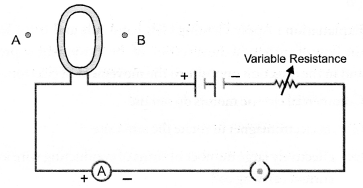(a).
Explanation : If current seems to flow in anticlockwise direction in a coil, then that face of the coil acts as N-pole.

Question 4.
In a long straight solenoid, N-and S-poles are created at the two ends of the solenoid. Which of the following statements is incor¬rect ?
(a) The field lines inside the solenoid are in the form of straight lines indicating that magnetic field is same at ail points inside the solenoid.
(b) The pattern of the magnetic field associated with the solenoid is different from the pattern of the magnetic field around a bar magnet.
(c) N-and S-poles exchange positions when the direction of the current through the solenoid is reversed.
(d) Magnetic field produced inside the solenoid can be used to magnetise a bar of magnetic material like soft iron, when placed inside the solenoid.
(b).
Explanation : The pattern of magnetic field produced by a cur¬rent carrying solenoid resembles with the pattern of magnetic field of a bar magnet.

Question 5.
A uniform magnetic field exists in the plane of paper pointing from left to right as shown in figure. In the field, an electron and a proton move as shown. The electron and proton experience(a) forces, both pointing into the plane of paper
(b) forces, both pointing out of the plane of paper
(c) forces, pointing into the plane of paper and out of the plane of paper respectively.
(d) forces, pointing opposite and along the direction of the uniform magnetic field respectively.
(a).
Explanation : Apply Fleming’s left hand rule and considering the flow of current in the direction of the movement of proton and in the direction opposite to the movement of electron.

Question 6.
Commercial electric motors do not use
(a) an electromagnet to rotate the armature
(b) effectively large number of turns of conducting wire in the current carrying coil
(c) a permanet magnet to rotate the armature id a soft iron on which the coil is wound.
(c).
Explanation : In commercial motor, armature rotates in the mag-netic field produced by an electromagnet. The strength of such magnetic field can be increased to the desired value. However, a permanent magnet provides a fixed and weak magnetic field.

Question 7.
Figure shows the arrangment of two coils wound on a non-conducting cylindrical rod. The plugh of the key is then in¬serted and later removed.(a) Deflection in the galvanometer remains zero throughout
(b) There is momentary deflection in the galvanometer that dies out shortly and deflections in both the cases are in the same direction
(c) There is momentary deflection in the galvanometer that dies out shortly and there is no effect when the key is re¬moved
(d) There are momentary deflections in both the cases that die out shortly but the deflections are in apposite directions.
(a).
Explanation : Due to electromagnetic induction, induced cur¬rent flows in the coil with which galvanometer is connected, when the key is inserted (i.e. current grows in the primary coil) and when the key is removed (i.e. current decays in the primary coil).

Question 8.
Choose the incorrect statement
(a) Fleming’s right hand rule is a simple rule to know the direction of induced current.
(b) The right-hand thumb rule is used to find the direction of magnetic fields due to current carrying conductors.
(c) The difference between the direct and alternating currents is that the direct current always flows in one direction, whereas the alternating current reverses its direction periodically.
(d) In India, the AC changes direction after every 1/60 seconds
(d).
Explanation : Frequency of AC in India = 50 Hz
∴ AC completes 1 cycle in 1/50 seconds.
In one cycle, AC changes direction two times. Hence, time after which AC changes directionQuestion 9.
A constant current flows in a horizontal wire in the plane of the paper from east to west as shown in figure. The direction of magnetic field at a point will be north to south.(a) directly below the wire
(b) directly above the wire
(c) at a point located in the plane of the paper, on the north side of the wire
(d) at a point located in the plane of the paper, on the south side of the wire.
(d).
Explanation : Applying right hand thumb rule to know the direction of the magnetic field.

Question 10.
The strength of magnetic field inside a long current carrying straight solenoid is
(a) more at the ends than at the centre
(b) minimum in the middle
(c) same at all points
(d) found to increase from one end to the other.
(c).
Explanation : Magnetic field inside a long current carrying straight solenoid is uniform.

Question 11.
To convert AC generator to DC generator
(a) slip rings and brushes must be used ‘
(b) split ring type commutator must be used
(c) rectangular wire loop is to be used
(d) stronger magnetic field is to be used.
(b).

Question 12.
The most important safety device method used for protecting electrical appliances from short circuiting or overloading is
(a) earthing
(b) use of stabilizers
(c) use of electric meter
(d) use of fuse.
(d).
Explanation : Fuse melts and the circuit breaks as soon as the current in the circuit exceeds safe limit.

Question 13.
A magnetic compass needle is placed in a plane of paper near point A as shown in figure.
In which plane should a straight current carrying conductor be placed so that it passes through A and there is no change in the deflection of the compass ? Under what condition is the deflection maximum and why?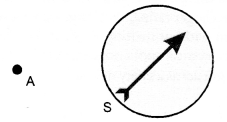The straight current carrying conductor should be placed in the plane of the paper. The deflection of the compass is maxi¬mum when the conductor passes through A and perpendicular to the plane of the paper. The magnetic field due to current carrying conductor is maximum in the plane of the paper.

Question 14.
Under what conditions permanent electromagnet is obtained if a current carrying solenoid is used ? Support your answer with the help of a labelled circuit diagram.
Permanent electromagnet is obtained if

1. direct current flows through the solenoid and
2. steel rod (a magnetic substance) is placed inside the solenoid.

In other words, a steel rod can be made a permanent electro-magnet if it is placed inside a solenoid carrying direct current as shown in figure.Question 15.
AB is a current carrying conductor in the plane of the paper as shown in figure. What are the directions of magnetic fields produced by it at points P and Q ? Given, r1 > r2, where will the strength of the magnetic field be larger ?
(CBSE 2010, 2012)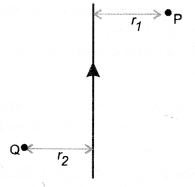According to Right hand thumb rule, the direction of the mag¬netic field is into the plane of the paper at P and the direction of the magnetic field is out of the plane of the paper at Q.
The strength of the magnetic field due to a current carrying conductor decreases with increase in distance (i.e. B ∝ 1/r ).
Therefore, strength of the magnetic field will be larger at point Q than at point P.

Question 16.
A magnetic compass shows a deflection when placed near a current carrying wire. How will the deflection of the compass get affected if the current in the wire is increased ? Support your answer with a reason. (CBSE 2012)
A magnetic compass is a tiny magnet. It gets deflected when placed in a magnetic field. When the current in the wire increases, then the magnetic field produced by the wire also increases ( B ∝ I). Hence, deflection of the compass increases.

Question 17.
It is established that an electric current through a metallic conductor produces a magnetic field around it. Is there a similar magnetic field produced around a thin beam of moving

1. alpha particles,
(CBSE 2012)

1. A thin beam of moving alpha particles constitute an electric current. Thus, beam of moving alpha particles behaves as a current carrying conductor. Hence, magnetic field is produced around the thin beam of moving alpha particles.
2. Neutrons are neutral particles i.e. they do not have any charge. Therefore, moving neutrons do not constitute an electric cur-rent. Hence, no magnetic field is produced around the thin beam of moving neutrons.

Question 18.
What does the direction of thumb indicate in the right-hand thumb rule. In what way this rule is different from Fleming’s left-hand rule ? (CBSE 2010,2012)
In the right hand rule, the direction of thumb indicates the direction of flow of current in the straight conductor.
Right-hand thumb rule is used to determine the direction of the magnetic field produced by a current carrying straight conductor. However, Flemings left hand rule is used to determine the direction of the force experienced by a current carrying conductor placed in a uniform magnetic field.

Question 19.
Meena draws magnetic field lines of field close to the axis of a current carrying circular loop. As she moves away from the centre of the circular loop, she observes that the lines keep on diverging. How will you explain her observation ? (CBSE 2010)
Magnetic field due to current carrying circular loop decreases as we move away from the centre of the loop. Diverging field lines represent weak magnetic field.

Question 20.
What does the divergence of magnetic field lines near the ends of a current carrying straight solenoid indicate ?
Divergence of magnetic field lines means the magnetic field lines are far apart. Such field lines indicate weak magnetic field. There¬fore, divergence of magnetic field lines near the ends of the current carrying solenoid indicates that the magnetic field near the ends of the current carrying solenoid is weak and non-uniform.

Question 21.
Name four appliances wherein an electric motor, a rotating device that converts electrical energy to mechanical energy is used as an important component. In what respect motors are different from generators ?
Appliances wherein an electric motor is used are

1. electric fan,
2. electric mixer,
3. washing machine and
4. a toy car.

Motor converts electrical energy into mechanical energy. How-ever, generator converts mechanical energy into electrical energy.

Question 22.
What is the role of two conducting stationary brushes in a simple electric motor ?
These brushes act as the contacts between the commutator and the terminals of the battery.

Question 23.
What is the difference between direct current and an alternating current ? How many times does AC used in India change direction in one second ?
Difference between Direct current and an Alternating current:

 Direct current Alternating current 1. The magnitude of direct current is constant and flows in one direction only.  2. Direct current cannot be used for large scale supply of electricity for household purpose. 3. The frequency of direct current is zero. 1. The magnitude of AC changes with time and direction of alternating current reverses periodically.  2. Alternating current is used to run electrical appliances like electric bulb, electric heater, electric iron, refrigerator etc. 3. Frequency of alternating current in India is 50 Hz.

Since frequency is 50 Hz, so a.c. completes 50 cycles in 1 second. Therefore, it reverses its direction 100 times in one second.

Question 24.
What is the role of fuse, used in series with any electrical appli-ances ? Why should a fuse with defined rating not be replaced by one with a larger rating ?
Electric fuse is a safety device. It protects the electrical appliance due to short circuiting and overloading in the electric circuit.
Fuse with defined rating means the maximum current that can flow’ through the fuse wire without melting it. As and when current exceeds this maximum value, the fuse melts and the circuit is switched off. If fuse with defined rating is replaced by one with a larger rating, then large current will flow through the circuit without melting the fuse. This large current may burn the electrical appliance connected in the circuit and the fuse will not act as a safety device.

## HOTS Questions for Class 10 Science Chapter 13 Magnetic Effects of Electric Current

Question 1.
One end of a bar magnet is brought near the south pole of a magnetic compass needle. It was noticed that the needle of the magnetic compass deflected away from the end of the bar magnet. Name the pole of the bar magnet at its end pointing towards the south pole of the magnetic compass. Justify your answer.
The pole of the bar magnet at the end pointing towards the south pole of the magnetic compass is south pole. This is because, like magnetic poles repel each other.

Question 2.
Magnetic field lines around a bar magnet are shown in figure. A student makes a statement that magnetic field at point A is stronger than at point B. State, whether the statement is correct or incorrect. Explain.
(CBSE 2012)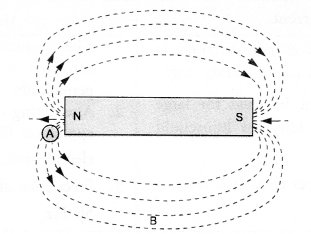Statement is correct. This is because field lines are crowded in a region of strong magnetic field and field lines diverge in a region of weak magnetic field.

Question 3.
Magnetic lines of force of two pairs of magnets are shown in figure A and B. Out of these two figures, which one represents the correct pattern of field lines. Name the poles of magnets facing each other. (CBSE 2012)Figure B represents the correct pattern of magnetic field lines of a pair of magnets. Figure A does not represent the correct pattern of field lines because magnetic field lines never cross each other.Poles of magnets facing each other are north poles as the magnetic field lines emerge from a magnet at N-pole.

Question 4.
A student performs an experiment to study the magnetic effect of current around a current carrying straight conductor. He reports that

1. the direction of deflection of the north pole of a compass needle kept at a given point near the conductor remains unaffected even when the terminals of the battery sending current in the wire are interchanged.
2. for a given battery, the degree of deflection of a N-pole decreases when the compass is kept at a point farther away from the conductor. Which of the above observations of the student is incorrect and why ?
(CBSE Sample Paper Question)

1. The first statement is incorrect because the direction of the magnetic field around the conductor is reversed when the direction of the current flowing in the conductor changes. Hence, the direction of deflection of N-pole of the compass needle cannot remain unaffected when the terminals of the battery sending current in the wire or conductor are interchanged.
2. The second statement is correct because the magnetic field decreases with the increase in the distance from the conductor.

Question 5.
A current carrying conductor is placed perpendicular to the magnetic field of horse-shoe magnet. The
conductor is displaced upward. What will happen to the displacement of the conductor if

1. current in the conductor is increased,
2. a horse-shoe magnet is replaced by another stronger horse-shoe magnet and
3. the length of the conductor is increased. (Similar Text Book Question)

1. The force acting on a current carrying conductor placed perpendicular to a magnetic field increases with the increase in the current flowing through a conductor. Thus, the displacement of the conductor will increase if the current in the conductor is increased.
2. When a horse-shoe magnet is replaced by a stronger magnet, then magnetic field increases. Since, force acting a conductor increases with the increase in the magnetic field, therefore, the displacement of the conductor will increase.
3. Since, the force acting on the conductor increases with the increases in the length of the conductor, therefore, the displacement of the conductor will increase.

## Value Based Questions in Science for Class 10 Chapter 13 Magnetic Effects of Electric Current

Question 1.
A fuse wire is a safety device to protect the electric circuit. Ashok used a fuse rated as 5 A in a circuit in which an electric heater of 2 kW was connected with the main supply of 220 V. The heater was not operating as the fuse wire melted on switching. Ashok was puzzled and he discussed the problem with his friend Ram. Ram suggeted him to use a fuse rated 10 A in the circuit. The problem of Ashok was solved as the heater was now working.
(i) What values are shown by Ram ?
(ii) How much current was flowing in the circuit with the fuse rated 10 A ?
(i) Helpful, high degree of awareness.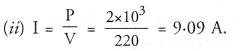Question 2.
Overloading in an electric circuit causes fire. Common people are unware of this fact. Akshil, an intelligent student told people of his locality about overloading. He told them to take various precautions to avoid overloading. What values are shown by Akshil ?
He is helpful and has high degree of awareness. Interested in educating people.

Question 3.
Students of a school ‘X’ went to a forest for a picnic. They went deep into the forest and started playing on a flat field in the forest. A stranger came there and asked for help. He had forgotten the direction of his village situated in south direction outside the forest. All the students except Sobit said that they had no idea about south direction. They asked stranger to find the south direction himself. However, Sobit asked him to wait for a moment. Sobit had a magnetic compass. He placed the magnetic compass on the ground and told the stranger about south direction.

1. How the magnetic compass determines the direction from the given place ?
2. What values are shown by Sobit ?
3. Is the behaviour of other students desirable ?

1. When a magnetic compass is placed at a place, it always points towards north-south direction.
2. Sobit is helpful, caring and has a high degree of awareness.
3. The behaviour of other students is undesirable. They did not bother about the problem of the stranger.

Question 4.
Aayan was going to his home from his school. On his way, he heard that people in a house were crying and weeping. He went to the house to know the reason. He found that a boy is lying on a floor and people around him were crying and weeping. He came to know that boy suffered a severe shock, when he was working with electric iron. He asked people to stop crying and help him to take the boy to the nearby dispensary for Medical aid. The boy was rushed to the dispensary. Medical aid was given to him and his life was saved.
Answer the following questions based on above information.

1. Why the boy suffered severe shock ?
2. Comment on the values shown by Aayan.

1. The boy might have touched the metallic casing of electric iron through which current was passing. Hence boy suffered a severe shock.
2. Aayan is helpful. He feels concerned about the welfare of others. He is quick in his actions and has high degree of general awareness.

Question 5.
What would you suggest to the people of your area regarding the safety measures in using electricity ?